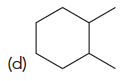Problem: Identify the number of stereoisomers expected for each of the following:

FREE Expert Solution
95% (101 ratings)
Problem Details

Identify the number of stereoisomers expected for each of the following:What scientific concept do you need to know in order to solve this problem?

Our tutors have indicated that to solve this problem you will need to apply the Determining Total Number of Stereoisomers concept. If you need more Determining Total Number of Stereoisomers practice, you can also practice Determining Total Number of Stereoisomers practice problems .

What is the difficulty of this problem?

Our tutors rated the difficulty of Identify the number of stereoisomers expected for each of th... as low difficulty.

How long does this problem take to solve?

Our expert Organic tutor, Jonathan took less than a minute to solve this problem. You can follow their steps in the video explanation above.

What textbook is this problem found in?

Our data indicates that this problem or a close variation was asked in . You can also practice practice problems .